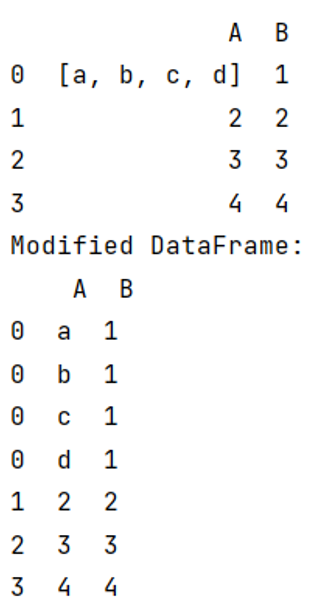# Split (explode) pandas DataFrame string entry to separate rows

Given a DataFrame, we have to split (explode) string entry to separate rows.
Submitted by Pranit Sharma, on May 13, 2022

Rows in pandas are the different cell (column) values that are aligned horizontally and also provide uniformity. Each row can have the same or different value. Rows are generally marked with the index number but in pandas, we can also assign index names according to the needs. In pandas, we can create, read, update and delete a column or row value.

Here, we are going to use pandas.DataFrame.explode() method.

Syntax:

```DataFrame.explode(
column,
ignore_index=False
)
```

To work with pandas, we need to import pandas package first, below is the syntax:

```import pandas as pd
```

Let us understand with the help of an example:

```# Importing Pandas package as pd
import pandas as pd

# Creating a dictionary
d = {'A':[['a','b','c','d'],2,3,4],'B':[1,2,3,4]}

# Creating DataFrame
df = pd.DataFrame(d)

# Display original DataFrame
print(df)

# Expanding a particular column
result = df.explode('A')

# Display Result
print("Modified DataFrame:\n",result)
```

Output:Languages: » C » C++ » C++ STL » Java » Data Structure » C#.Net » Android » Kotlin » SQL
Web Technologies: » PHP » Python » JavaScript » CSS » Ajax » Node.js » Web programming/HTML
Solved programs: » C » C++ » DS » Java » C#
Aptitude que. & ans.: » C » C++ » Java » DBMS
Interview que. & ans.: » C » Embedded C » Java » SEO » HR
CS Subjects: » CS Basics » O.S. » Networks » DBMS » Embedded Systems » Cloud Computing
» Machine learning » CS Organizations » Linux » DOS
More: » Articles » Puzzles » News/Updates